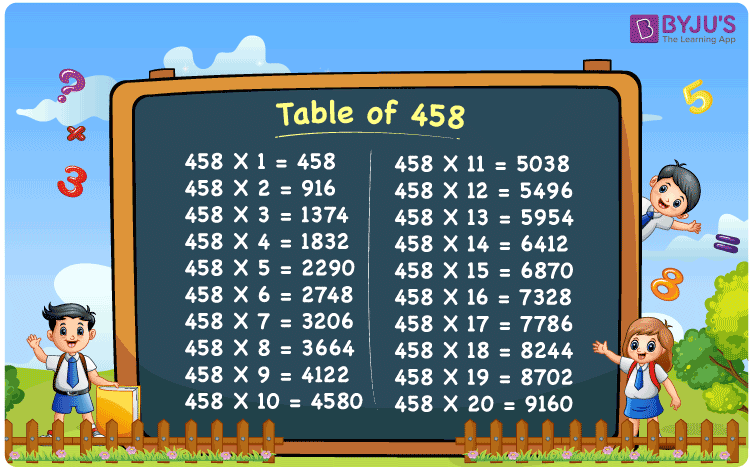Checkout JEE MAINS 2022 Question Paper Analysis : Checkout JEE MAINS 2022 Question Paper Analysis :

# Table of 458

The Table of 458 is provided in a tabular format to help students quickly check the values of multiples of 458. The multiplication table of 458 up to 20 is also available in PDF format to download, which will be handy while solving problems related to the 458 times table. The table of 458 would help do mental maths, which is a vital skill for appearing in any competitive examination.

## Table of 458 Chart

The 458 times table chart up to 20 is given here with an attractive image that can be used as a flashcard to memorise the times table of 458. The Multiplication table of 458 will help students go through the multiples of 458.## What is the 458 Times Table?

The 458 times table is the multiplication of 458 with any natural number. The 458 times table can be obtained by multiplication and by adding 458 repeatedly. The below chart shows the multiplication table of 458, in both multiplication and addition form.

 458×1 = 458 458 458×2 = 916 458 + 458 = 916 458×3 = 1374 458 + 458 + 458 = 1374 458×4 = 1832 458 + 458 + 458 + 458 = 1832 458×5 = 2290 458 + 458 + 458 + 458 + 458 = 2290 458×6 = 2748 458 + 458 + 458 + 458 + 458 + 458 = 2748 458×7 = 3206 458 + 458 + 458 + 458 + 458 + 458 + 458 = 3206 458×8 = 3664 458 + 458 + 458 + 458 + 458 + 458 + 458 + 458 = 3664 458×9 = 4122 458 + 458 + 458 + 458 + 458 + 458 + 458 + 458 + 458 = 4122 458×10 = 4580 458 + 458 + 458 + 458 + 458 + 458 + 458 + 458 + 458 + 458 = 4580

## Multiplication Table of 458

Given below are the first 20 multiples of 458.

 458 × 1 = 458 458 × 2 = 916 458 × 3 = 1374 458 × 4 = 1832 458 × 5 = 2290 458 × 6 = 2748 458 × 7 = 3206 458 × 8 = 3664 458 × 9 = 4122 458 × 10 = 4580 458 × 11 = 5038 458 × 12 = 5496 458 × 13 = 5954 458 × 14 = 6412 458 × 15 = 6870 458 × 16 = 7328 458 × 17 = 7786 458 × 18 = 8244 458 × 19 = 8702 458 × 20 = 9160

## Solved Example on the Table of 458

Example 1:

What is two more than the third multiple of 458?

Solution:

The third multiple of 458 = 458×3 = 1374

Two more than 1374 = 1374 + 2 = 1376.

## Frequently Asked Questions on the Table of 458

### What is the times tables of 458?

The 458 times table is the multiplication of 458 with natural numbers. Starting with 458 × 1 = 458, 458 × 2 = 916, 458 × 3 = 1374, 458 × 4 = 1832, 458 × 5 = 2290 and so on.

### What times table has 458?

458 comes in times tables of 1, 2, 229 and 458.

### What is 15 times 458?

15 times 458 is 6870.